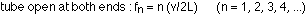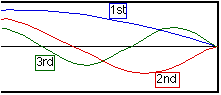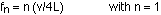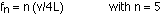## The physics of music

3-17-99

Sections 12.5 - 12.7

The physics behind musical instruments is beautifully simple. The sounds made by musical instruments are possible because of standing waves, which come from the constructive interference between waves traveling in both directions along a string or a tube..

### How a guitar works

A typical guitar has six strings. These are all of the same length, and all under about the same tension, so why do they put out sound of different frequency? If you look at the different strings, they're of different sizes, so the mass/length of all the strings is different. The one at the bottom has the smallest mass/length, so it has the highest frequency. The strings increase in mass/length as you move up, so the top string, the heaviest, has the lowest frequency.

Tuning a guitar simply means setting the fundamental frequency of each string to the correct value. This is done by adjusting the tension in each string. If the tension is increased, the fundamental frequency increases; if the tension is reduced the frequency will decrease.

To obtain different notes (i.e., different frequencies) from a string, the string's length is changed by pressing the string down until it touches a fret. This shortens a string, and the frequency will be increased.

### Wind instruments and longitudinal standing waves

Pipes work in a similar way as strings, so we can analyze everything from organ pipes to flutes to trumpets. The big difference between pipes and strings is that while we consider strings to be fixed at both ends, the tube is either free at both ends (if it is open at both ends) or is free at one end and fixed at the other (if the tube is closed at one end). In these cases the harmonic frequencies are given by:A pipe organ has an array of different pipes of varying lengths, some open-ended and some closed at one end. Each pipe corresponds to a different fundamental frequency. For an instrument like a flute, on the other hand, there is only a single pipe. Holes can be opened along the flute to reduce the effective length, thereby increasing the frequency. In a trumpet, valves are used to make the air travel through different sections of the trumpet, changing its effective length; with a trombone, the change in length is a little more obvious.

Example : A tube open at one end has a length of 25.0 cm. The temperature is 20 °C. What is the fundamental frequency of this tube? What is the frequency of the fifth harmonic?

If we blow through the tube, it will make a musical tone, and that's what we're talking about here. The velocity involved in the frequency equation is therefore the speed of sound, which is 343 m/s at 20 °C. The fundamental frequency is then:So the fundamental is 343 / ( 4 x 0.25) = 343 Hz.

A tube like this, closed at one end, only has odd harmonics (n = 1, 3, 5, etc.). The fifth harmonic is five times the fundamental, and it's also given by:So the fifth harmonic is 1715 Hz.

### Beats

When two waves which are of slightly different frequency interfere, the interference cycles from constructive to destructive and back again. This is known as beats; two sound waves producing beats will generate a sound with an intensity that continually cycles from loud to soft and back again. The frequency of the sound you hear will be the average of the frequency of the two waves; the intensity will vary with a frequency (known as the beat frequency) that is the difference between the frequencies of the two waves.